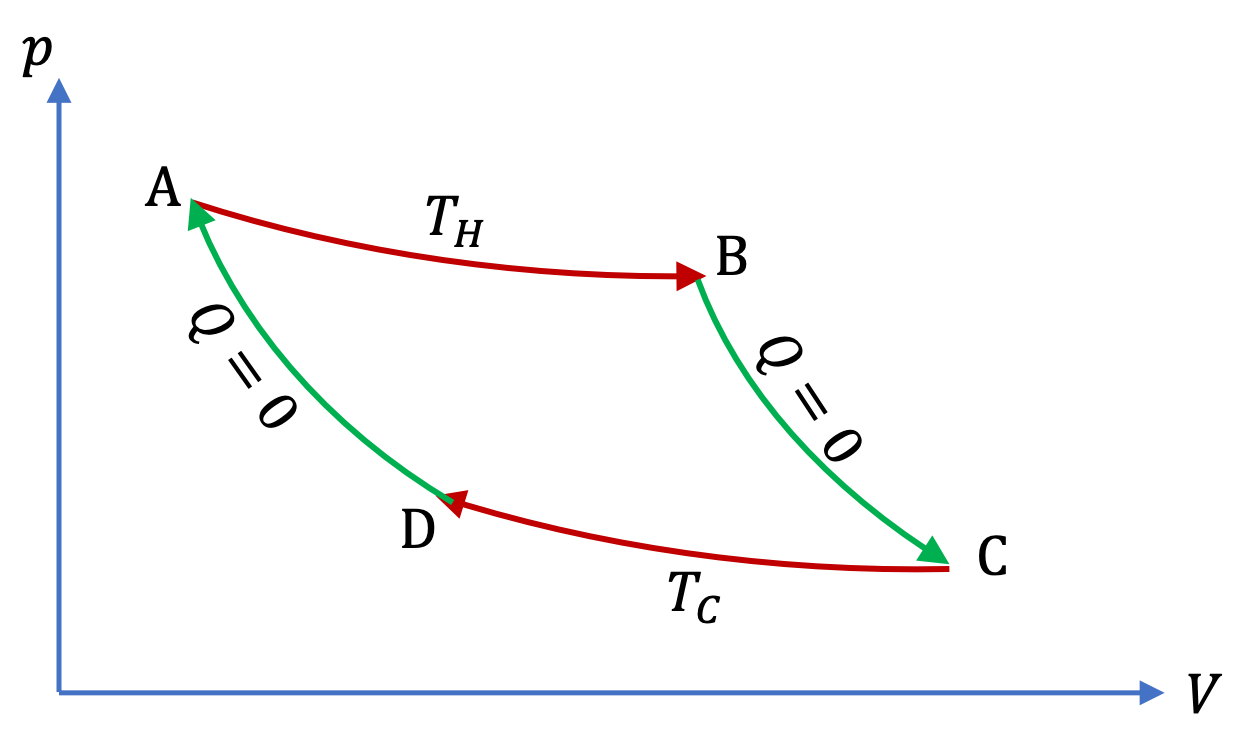## Section26.1Reversible Process

How would you heat a tank of gas so slowly that at each instant it remains in equilibrium? This is clearly not possible in reality. In order for heat to flow into the gas, you would need environment of the gas to be at a higher temperature than the gas. This would make the gas near wall to be at a slightly higher temperature than the rest of the gas. This means that the gas would not be in thermal equilibrium with itself or with the environment.

However, we can imagine an ideal process in which the difference is negligible. Say, the temperature of the gas is $300\text{K}\text{.}$ If we place the tank in a large water bath also at temperarture $300\text{K}\text{,}$ then gas and the water will be in thermal equilibrium. Now, if we increase the temperature of the water bath ever so sligtly, heat will flow in the gas but we can control the heating rate so that if we were to cool the water a little bit, the change will be exactly reversed restoring the original states of everything. This can be guaranteed to a degree of accuracy we may demand, especially if this is done in infinitely many infinitesimal steps.

The ideal slow process described in the last paragraph is called reversible process, since reversing the previous infintesimal step completely restores all states.

Every step of a Carnot cycle is a reversible process. It was shown by Carnot that the heat exchanged by the gas in a Carnot cycle obeys a proportionality relation to the temperature of the two baths.Carnot showed that heat $Q_H$ absorbed by the ideal gas at temperature $T_H$ and heat $Q_C$ released at the lower temperature $T_C$ are in the same proportion as the temperatures expressed in the absolute scale.

\begin{equation*} \left| \dfrac{Q_H}{Q_C} \right| = \dfrac{T_H}{T_C} \end{equation*}

Using a positive sign for an energy entering the system and a negative for the energy leaving the system, we obtain the following over a cycle.

\begin{equation*} \dfrac{Q_H}{-Q_C} = \dfrac{T_H}{T_C} \end{equation*}

Rearranging this equation, we find the following relation for the heat exchange of the Carnot engine with the environment over a cycle.

\begin{equation} \frac{Q_H}{T_H} + \frac{Q_C}{T_C} = 0.\label{eq-qh-th-qc-tc-carnot}\tag{26.1.1} \end{equation}

Suppose you think of the isothermal step of heating at $T_H$ as made up tiny steps each with heat $\Delta Q$ at $T_H\text{.}$ And similarly for the processes at the colder bath. That would mean Eq. (26.1.1) is actually a n infinte sum of infinitesimal steps. By doing that we get an very general result, applicable not only to the Carnot cycle but to every reversible cyclic process.

\begin{equation} \sum{\frac{\Delta Q}{T}} = 0.\ \ \ \ \text{(Reversible Cyclic Process)}\label{eq-carnot-cycle-heat-1}\tag{26.1.2} \end{equation}

This more general conclusion was obtained mathematically by Clausius. He actually obtained an inequality that is obeyed by all cyclic processes, where equality is for the reversible process.

\begin{equation} \sum\frac{\Delta Q}{T} \le 0 \ \ \ \textrm{(All cycle processes in nature)}.\label{eq-entropy-inequality-delta-form}\tag{26.1.3} \end{equation}